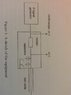# Stray/Parasitic Capacitance - Impacts phase velocity?

Strides
Hey everyone,

I'm trying to measure the stray capacitance in a circuit comprised of an rf signal generator, an oscilloscope, a coaxial cable (short-circuited) and some capacitors. I measured the resonant frequencies of the coaxil cable for varying values of capacity (0pF to 850pF) between 4MHz and 100MHz. I then used the following equation to plot X vs capacity and find the corresponding characteristic impedance and stray capacitance:

$$X = ZC_{x} + ZC_{s}$$

Where X is:

$$X = 1/(2πf tan(2πfl/v_p))$$

(I was only given the function for X, so not entirely sure what it describes, I'll be grateful to anyone who is able to offer some insight or background theory)

l = length of test cable, 2m
f = frequency
v = phase velocity

Results from graph:

Z = 55.167
Cs = 29.5pF

Does this value seem sensible for the given frequency/capacitance range?

I then calculated the phase velocity of the coaxil cable to be around 1.94*10^(-8), and that the stray capacitance increases the phase velocity by around a mere 7m/s. Again is this expected for the given range?

Thanks for all the help, in advance.

Last edited:

Mentor
Can you upload a picture of your setup, and a schematic diagram?

29pF is a little high for parasitic capacitance, but it's hard to say without understanding your setup better.

Strides
At the moment, all I've got is a simple diagram, I hope that suffices.

#### Attachments

•Setup diagram.jpg
25.6 KB · Views: 442
Mentor
And you want to measure which parasitic capacitance? On the far side of the resistor where the cable is (minus the expected cable capacitance)? What is the capacitance of your 'scope probes?

Strides
The scope probes are just coaxil cables connected to the oscilloscope, which should be around 50Ω & 90pF per meter. With a length of a couple cm, I assume roughly 2pF per channel?

I was hoping to measure any parasitic capacitance which may effect the phase velocity. I was only given the above equation in the experiment to measure it by, so I'm unsure of primarily where that would be based in the circuit. I would be grateful for any explanation that you're willing to give regarding that.

Gold Member
X=ZCx+ZCsX=ZCx+ZCs​
That would apply for a series stray C. For parallel C, you would need the Admittance equivalent.

Strides
I've been specifically told to use the equation above for the serial stray capacitance, so I assume that in this experiment the parallel stray capacitance that you've mentioned must be taken as negligible.

Strides
I mainly just want to know, if the increase in the phase velocity for the given stray capacitance is an acceptable value for the given range of frequency?

Gold Member
I've been specifically told to use the equation above for the serial stray capacitance, so I assume that in this experiment the parallel stray capacitance that you've mentioned must be taken as negligible.
The C's in your diagram are in parallel. I wonder what serial capacitance is suggested - a capacitative coupling perhaps. In any case that is not mentioned on the diagram. Perhaps you should check or ask for advice from the guy who set the question?
I'm not sure of the context of the question. Are you finding the phase velocity from the electrical length of the transmission line and the Impedance? The additional (parallel) C 's will modify the electrical length.
I don't imagine you would be using a Smith Chart to solve the problem these days but the graphical method is great for getting to understand what is going on.

Strides
Here's the lab guide that I've been using, the question for stray capacitance is at 7.4. I've already found the phase velocity from the length of the transmission line without taking the stray capacitance into consideration.

#### Attachments

• RF_Lines.pdf
192.8 KB · Views: 364